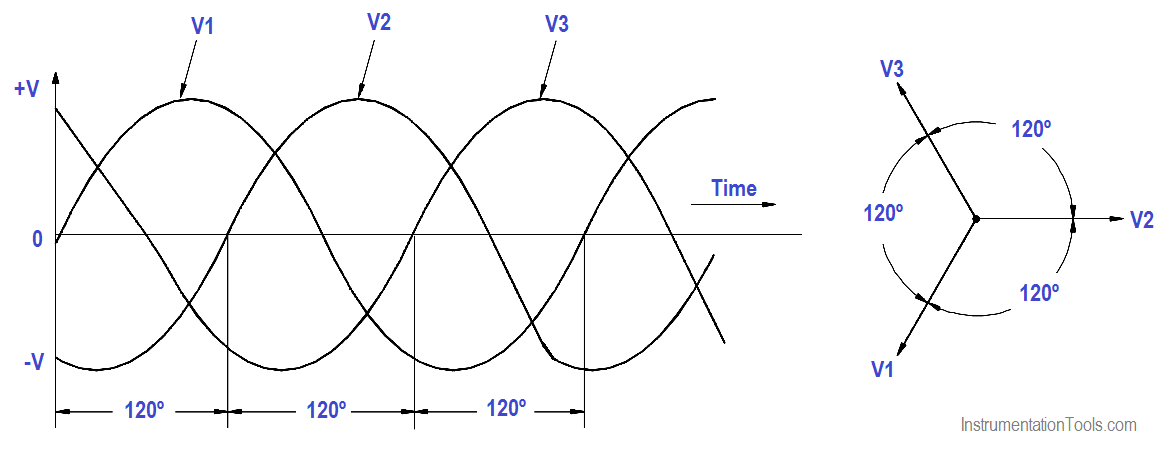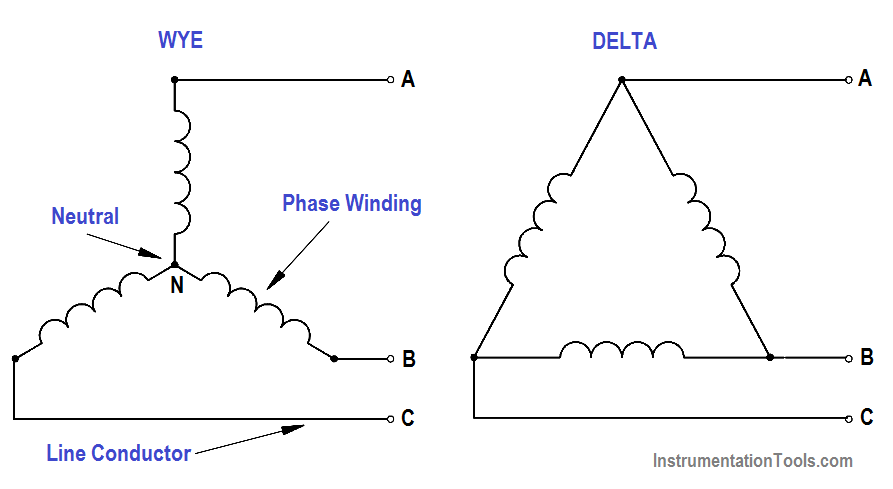# Three Phase Systems

A three-phase (3φ) system is a combination of three single-phase systems. In a 3φ balanced system, power comes from a 3φ AC generator that produces three separate and equal voltages, each of which is 120° out of phase with the other voltages (Figure 10).Figure 10 : Three-Phase AC

Three-phase equipment (motors, transformers, etc.) weighs less than single-phase equipment of the same power rating. They have a wide range of voltages and can be used for single-phase loads. Three-phase equipment is smaller in size, weighs less, and is more efficient than single-phase equipment.

Three-phase systems can be connected in two different ways. If the three common ends of each phase are connected at a common point and the other three ends are connected to a 3φ line, it is called a wye, or Y, connection (Figure 11). If the three phases are connected in series to form a closed loop, it is called a delta, or ∆, connection.Figure 11 : 3φ AC Power Connections